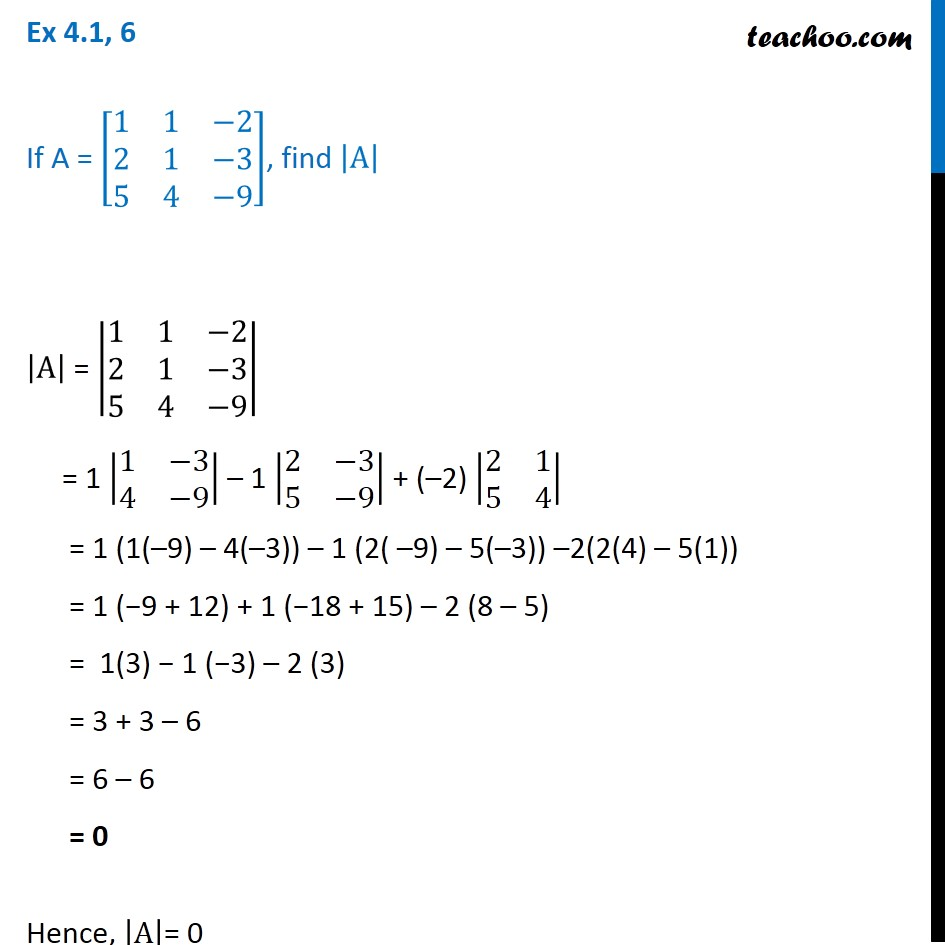1. Chapter 4 Class 12 Determinants (Term 1)
2. Serial order wise
3. Ex 4.1

Transcript

Ex 4.1, 6 If A = [■8(1&1&−2@2&1&−3@5&4&−9)], find |A| |A| = |■8(1&1&−2@2&1&−3@5&4&−9)| = 1 |■8(1&−3@4&−9)| – 1 |■8(2&−3@5&−9)| + (–2) |■8(2&1@5&4)| = 1 (1(–9) – 4(–3)) – 1 (2( –9) – 5(–3)) –2(2(4) – 5(1)) = 1 (−9 + 12) + 1 (−18 + 15) – 2 (8 – 5) = 1(3) − 1 (−3) – 2 (3) = 3 + 3 – 6 = 6 – 6 = 0 Hence, |A|= 0

Ex 4.1

Chapter 4 Class 12 Determinants (Term 1)
Serial order wise